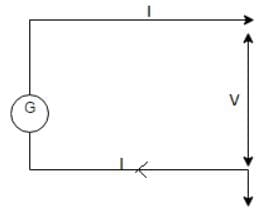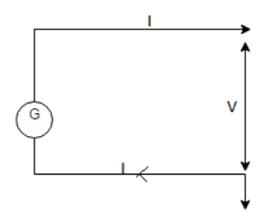Test: Distribution System

# Test: Distribution System

Test Description

## 10 Questions MCQ Test Power Systems | Test: Distribution System

Test: Distribution System for Electrical Engineering (EE) 2023 is part of Power Systems preparation. The Test: Distribution System questions and answers have been prepared according to the Electrical Engineering (EE) exam syllabus.The Test: Distribution System MCQs are made for Electrical Engineering (EE) 2023 Exam. Find important definitions, questions, notes, meanings, examples, exercises, MCQs and online tests for Test: Distribution System below.
Solutions of Test: Distribution System questions in English are available as part of our Power Systems for Electrical Engineering (EE) & Test: Distribution System solutions in Hindi for Power Systems course. Download more important topics, notes, lectures and mock test series for Electrical Engineering (EE) Exam by signing up for free. Attempt Test: Distribution System | 10 questions in 30 minutes | Mock test for Electrical Engineering (EE) preparation | Free important questions MCQ to study Power Systems for Electrical Engineering (EE) Exam | Download free PDF with solutions
 1 Crore+ students have signed up on EduRev. Have you?
Test: Distribution System - Question 1

### A DC 2-wire system with mid-point earthed having cross-sectional area of each conductor be ‘a’ and resistance ‘R1’. If the DC tow wire replaces the system, then the ratio of volume of each conductor V1/V2 will be ___________

Detailed Solution for Test: Distribution System - Question 1

Let the power losses remain constant over the systems, then
2P2R/V2 = 2P2R1/2*V2
or, R = R1/4;
As R = lρ/a;
a1 = a/4;
So, V1/V1 = 1/4.

Test: Distribution System - Question 2

### A DC 2-wire system with mid-point earthed having cross-sectional area of each conductor be ‘a’ and resistance ‘R1’. If this is replaced by monopolar system, then saving of copper will be ______

Detailed Solution for Test: Distribution System - Question 2

Let the power losses remain constant over the systems, then
2P2R/V2 = 2P2R1/V2
or, R1 = R
As R = lρ/a;
a1 = a
So, V1/V1 = a* 2l/a1 = 1/2.

Test: Distribution System - Question 3

### For the given distribution system the maximum voltage at the midpoint will be ________

Detailed Solution for Test: Distribution System - Question 3

Vmax = V/√2 = (440/2)/√2 = 155.6 V.

Test: Distribution System - Question 4

A transmission and distribution engineer needed to design the sub transmission substation. The tapping component needed will be ______.

Detailed Solution for Test: Distribution System - Question 4

For the sub transmission level, there is no tapping at various level does not take place. For this a feeder is used.

Test: Distribution System - Question 5

A _________ distribution system is more reliable than the ______ distribution system.

Detailed Solution for Test: Distribution System - Question 5

A parallel distribution system has two end feeding and an alternative of parallel line, so in case there is a fault, the isolator can isolate the faulty part and let the healthy system operate.

Test: Distribution System - Question 6

For the figure shown, having a 2-wire dc system where mid point is unearthed, having resistance of 20 ohms, with a power transmitted of 5 MW at the voltage level of 440 kV.
The power loss of the distribution system will be ________.Detailed Solution for Test: Distribution System - Question 6

I = P/V = 5000000/440000 = 11.36 A
Power loss = I2 * R = 2 * 11.362 * 20 = 2583 W.

Test: Distribution System - Question 7

While designing the distribution sub stations by the designer, it is required to use the _______ for the discrete power tapping.

Detailed Solution for Test: Distribution System - Question 7

For the discrete tapping of the power the consumer level it is the distributor which is employed at the sub stations and consumer levels.

Test: Distribution System - Question 8

For the single phase 2-wire system, the maximum voltage between the outer wires and earth is ________

Detailed Solution for Test: Distribution System - Question 8

The midpoint voltage will be V/√2.

Test: Distribution System - Question 9

For the figure shown, having a 2-wire dc system where mid point is earthed, having resistance of 20 ohms, with a power transmitted of 5 MW at the voltage level of 440 kV.
The power loss of the distribution system will be __________Detailed Solution for Test: Distribution System - Question 9

I = P/V = 5000000/440000 = 11.36 A
Power loss = 2I2 * R = 2 * 11.362 * 20 = 5165 W.

Test: Distribution System - Question 10

While designing the distribution to locality of one lac population with medium dense load requirement, we can employ ________.

Detailed Solution for Test: Distribution System - Question 10

A radial system is used for the medium requirement of loading and a medium dense population as it becomes easier to install and operate.

## Power Systems

42 docs|34 tests
 Use Code STAYHOME200 and get INR 200 additional OFF Use Coupon Code
Information about Test: Distribution System Page
In this test you can find the Exam questions for Test: Distribution System solved & explained in the simplest way possible. Besides giving Questions and answers for Test: Distribution System, EduRev gives you an ample number of Online tests for practice

42 docs|34 tests